# Abstract polytope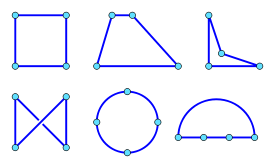As abstract polytopes, these quadrilaterals are all the same.

In mathematics, an abstract polytope, informally speaking, is a structure which considers only the combinatorial properties of a traditional polytope, ignoring many of its other properties, such as angles, edge lengths, etc. The abstract formulation embodies the combinatorial properties as a partially ordered set or poset.

No space, such as Euclidean space, is required to contain it. An ordinary geometric polytope is said to be a realization of the corresponding abstract polytope.

The abstract definition allows some more general combinatorial structures than the traditional concept of a polytope, and allows many new objects that have no counterpart in traditional theory.

The term polytope is a generalisation of polygons and polyhedra into any number of dimensions.

In Euclidean geometry, the six quadrilaterals above are all different. Yet they have a common structure that is not shared by a triangle or a cube, for example.

Considering each vertex, edge, etc. as a distinct element of the polytope, in each of the above examples the connections or incidences between elements are the same, regardless of the physical layout. The objects are said to be combinatorially equivalent. This equivalence is what is encapsulated in the concept of an abstract polytope. So, combinatorially, our six quadrilaterals are all the “same”. More rigorously, they are said to be isomorphic or “structure preserving”.

The measurable properties of traditional polytopes such as angles, edge-lengths, skewness, straightness and convexity have no meaning for an abstract polytope. Other traditional concepts may carry over, but not always identically. What is true for traditional polytopes may not be so for abstract ones, and vice versa. For example, a traditional polytope is regular if all its facets and vertex figures are regular, but this is not so for abstract polytopes.

## Introductory concepts

To define an abstract polytope, a few preliminary concepts are needed.

Throughout this article, polytope means abstract polytope - unless stated otherwise. The term traditional will be used, somewhat loosely, to refer to what is generally understood by polytope, excluding our abstract polytopes. Some authors also use the terms classical or geometric.

### Polytopes as posets

The connections on a railway map or electrical circuit can be represented quite satisfactorily with just “dots and lines” - i.e. a graph. Polytopes, however, have a dimensional hierarchy. For example, the vertices, edges and faces of a cube have dimension 0, 1, and 2 respectively; the cube itself is 3-dimensional.

In our abstract theory, the concept of rank replaces that of dimension; we formally define it below.

We use the term face to refer to an element of any rank, e.g. vertices (rank 0) or edges (rank 1), and not just faces of rank 2. An element of rank k is called a k-face.

We shall define a polytope, then, as a set of faces P with an order relation <, and which satisfies certain additional axioms. Formally, P (with <) will be a (strict) partially ordered set, or poset.

When F < G, we say that F is a subface of G (or G has subface F).

We say F, G are incident if either F = G or F < G or G < F. This meaning differs from its usage in traditional geometry and some other areas of mathematics. For example, in the square abcd, edges ab and bc are not incident. But it is the same notion of incidence as used in Finite geometry.

### Least and greatest faces

Just as the concepts of zero and infinity are indispensable in mathematics, it turns out to be extremely useful and elegant, indeed essential, to insist that every polytope also has a least face, which is a subface of all the others, and a greatest face, of which all the others are subfaces.

In fact, a polytope can have just one face; in this case the least and greatest faces are one and the same.

The least and greatest faces are called improper faces; all others faces are proper faces.

The least face is called the null face, since it has no vertices (or any other faces) as subfaces. Since the least face is one level below the vertices or 0-faces, its rank is −1; we often denote it as F−1. If this seems strange at first, the feeling is quickly dispelled on seeing the elegant symmetry which this concept brings to our theory. (Historically, mathematicians resisted such commonplace concepts as negative, fractional, irrational and complex numbers - and even zero!)

### A simple example

As an example, we now create an abstract square, which has faces as in the table below:

Face type Rank (k) Count k-faces
Least −1 1F−1
Vertex 0 4a, b, c, d
Edge 1 4W, X, Y, Z
Greatest 2 1G

The relation < is defined as set of pairs, which (for this example) would include

F−1<a, ... , F−1<X, ... , F−1<G, ... , b<Y, ... , c<G, ... , Z<G.

In this particular example, we could have written the edges W, X, Y and Z as ab, ad, bc, and cd respectively, and we often will use this vertex notation. But as we shall shortly see, such notation is not always appropriate.

We have called this a square rather than a quadrilateral (or tetragon) because, in our abstract world, there are no angles, and edges do not have lengths. All four edges are identical, and the "geometry" at each vertex is the same.

Order relations are transitive, i.e. F < G and G < H implies that F < H. Therefore, to specify the hierarchy of faces, it is not necessary to give every case of F < H, only the pairs where one is the successor of the other, i.e. where F < H and no G satisfies F < G < H.

### The Hasse diagram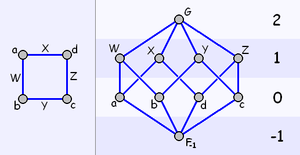The graph (left) and Hasse diagram of a square, showing ranks (right)

Smaller posets, and polytopes in particular, are often best visualised by using a Hasse diagram, as shown. By convention, faces of the equal rank are placed on the same vertical level. Each "line" between faces indicates a pair F, G such that F < G where F is below G in the diagram.

A polytope is often depicted informally by its graph, but the two cannot be equated. A graph has vertices and edges, but no other faces. Furthermore, for most polytopes, it is not possible to deduce all the other faces from the graph, and, in general, different polytopes can have the same graph.

A Hasse diagram, on the other hand, fully describes any poset - all the structure of the polytope is captured in the Hasse diagram. Isomorphic polytopes give rise to isomorphic Hasse diagrams, and vice versa.

### Rank

The rank of a face F is defined as the integer (m  2), where m is the maximum number of faces in any chain (F', F", ... , F) satisfying F' < F" < ... < F.

The rank of a poset P is the maximum rank n of any face, i.e. that of the greatest face (given that we require that there is one). Throughout this article, we shall always use n to denote the rank of the poset or polytope under discussion.

It follows that the least face, and no other, has rank −1; and that the greatest face has rank n. We often denote these as F−1 and Fn respectively.

The rank of a face or polytope usually corresponds to the dimension of its counterpart in traditional theory - but not always. For example, a face of rank 1 corresponds to an edge, which is 1-dimensional. But a skew polygon in traditional geometry is 3-dimensional, since it is not flat (planar); while its abstract equivalent, and indeed all abstract polygons, have rank 2.

For some ranks, we have names for their face-types, as in the table.

Rank -1 0 1 2 3 ... n - 2 n - 1 n
Face Type Least Vertex Edge Cell Subfacet or ridge Facet Greatest

† Although traditionally "face" has meant a rank 2 face, we shall always write "2-face" to avoid ambiguity, reserving the term "face" to mean a face of any rank.

### The line segment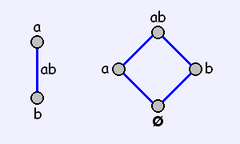The graph (left) and Hasse Diagram of a line segment

A line segment is a poset that has a least face, precisely two 0-faces, and a greatest face, for example {ø, a, b, ab}. It follows easily that the vertices a and b have rank 0, and that the greatest face ab, and therefore the poset, both have rank 1.

### Flags

A flag is a maximal chain of faces, i.e. a (totally) ordered set Ψ of faces, each a subface of the next (if any), and such that Ψ is not a subset of any larger chain.

For example, {ø, a, ab, abc} is a flag in the triangle abc.

We shall additionally require that, for a given polytope, all flags contain the same number of faces. Posets do not, in general, satisfy this requirement; the poset {ø, a, b, bc, abc} has 2 flags of unequal size, and is not therefore a polytope.

Clearly, given any two distinct faces F, G in a flag, either F < G or F > G.

### Sections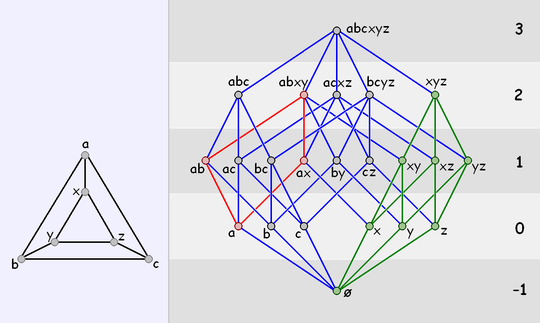The graph (left) and Hasse Diagram of a triangular prism, showing a 1-section (red), and a 2-section (green).

Any subset P' of a poset P is a poset (with the same relation <, restricted to P').

In particular, given any two faces F, H of P with FH, the set {G | FGH} is called a section of P, and denoted H/F. (In order theory terminology, a section is called a closed interval of the poset and denoted [F, H], but the concepts are identical).

P is thus a section of itself.

For example, in the prism abcxyz (see Figure) the section xyz/ø (highlighted green) is the triangle

{ø, x, y, z, xy, xz, yz, xyz}.

A k-section is a section of rank k.

A polytope that is the subset of another polytope is not necessarily a section. The square abcd is a subset of the tetrahedron abcd, but is not a section of it.

This concept of section does not have the same meaning as in traditional geometry.

#### Vertex figures

The vertex figure at a given vertex V is the (n1)-section Fn/V, where Fn is the greatest face.

For example, in the triangle abc, the vertex figure at b, abc/b, is {b, ab, bc, abc}, which is a line segment. The vertex figures of a cube are triangles.

#### Connectedness

A poset P is connected if P has rank ≤ 1, or, given any two proper faces F and G, there is a sequence of proper faces

H1, H2, ... ,Hk

such that F = H1, G = Hk, and each Hi, i < k, is incident with its successor.

The above condition ensures that a pair of disjoint triangles abc and xyz is not a (single) polytope.

A poset P is strongly connected if every section of P (including P itself) is connected.

With this additional requirement, two pyramids that share just a vertex are also excluded. However, two square pyramids, for example, can, be "glued" at their square faces - giving an octahedron. The "common face" is not then a face of the octahedron.

## Formal definition

An abstract polytope is a partially ordered set, whose elements we call faces, satisfying the 4 axioms:

1. It has a least face and a greatest face.
2. All flags contain the same number of faces.
3. It is strongly connected.
4. Every 1-section is a line segment.

An n-polytope is a polytope of rank n.

### Notes

In the case of the null polytope, the least and greatest faces are the same single element.

Axiom 2 is equivalent to saying that the poset is a graded poset.

Given the other axioms, Axiom 3 is equivalent to strong flag-connectedness, which informally means:

For any section of the polytope (including the polytope itself), any flag can be changed into any other by changing just one face at a time.

Axiom 4 is known as the “diamond property”, since the Hasse Diagram of a line segment is diamond-shaped.

It can be shown from the axioms that every section is a polytope, and that Rank(G/F) = Rank(G) − Rank(F) − 1.

## The simplest polytopes

### Rank < 2

There is just one polytope for each rank −1, 0 and 1, and these are, respectively, the null polytope, the point, and the line segment.

For n ≤ 1, all n-sections of a polytope are the (unique) n-polytope. However, faces of rank 0 and 1 of a polytope are called vertices and edges respectively.

### Rank 2

For each p, 3 ≤ p <, we have the (abstract equivalent of) the traditional polygon with p vertices and p edges, or a p-gon. For p = 3, 4, 5, ... we have the triangle, square, pentagon, ....

For p = 2, we have the digon, and p =we get the apeirogon.

#### The digon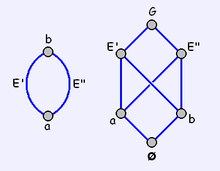The graph (left) and Hasse Diagram of a digon

A digon is a polygon with just 2 edges. Unlike any other polygon, both edges have the same two vertices. For this reason, it is degenerate in the Euclidean plane.

Until now, we have defined face sets using "vertex notation" - e.g. {ø, a, b, c, ab, ac, bc, abc} for the triangle abc. This method has the decided advantage of implying the < relation.

With the digon vertex notation cannot be used. We are forced to give the faces individual symbols and specify the subface pairs F < G.

Thus a digon must be defined as a set {ø, a, b, E', E", G} with the relation < given by

{ø<a, ø<b, a<E', a<E", b<E', b<E", E'<G, E"<G}

where E' and E" are the two edges, and G the greatest face.

This need to identify each element of the polytope with a unique symbol apples to many other abstract polytopes and is therefore common practice.

A polytope can only be fully described using vertex notation if every face is incident with a unique set of vertices. A polytope having this property is said to be atomistic.

## Examples of higher rank

As stated above, this concept of an abstract polytope is very general, and includes:

• Apeirotopes, i.e. infinite polytopes or tessellations (tilings)
• Decompositions of other manifolds such as the torus or real projective plane
• Many other objects, such as the 11-cell and the 57-cell, that don't fit well into "normal" geometric spaces.

In general, the set of j-faces (−1 ≤ jn) of a traditional n-polytope form an abstract n-polytope.

### Hosohedra and hosotopes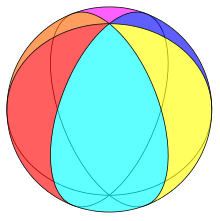A hexagonal hosohedron, realized as a spherical polyhedron.

The digon is generalized by the hosohedron and higher dimensional hosotopes, which can all be realized as spherical polyhedra – they tessellate the sphere.

### Projective polytopes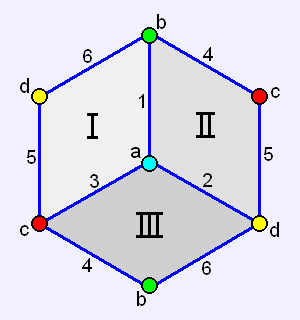The Hemicube is derived from a cube by equating opposite vertices, edges, and faces. It has 4 vertices, 6 edges, and 3 faces.

Four examples of non-traditional abstract polyhedra are the Hemicube (shown), Hemi-octahedron, Hemi-dodecahedron, and the Hemi-icosahedron. These are the projective counterparts of the Platonic solids, and can be realized as (globally) projective polyhedra – they tessellate the real projective plane.

The hemicube is another example of where vertex notation can't be used to define a polytope - all the 2-faces and the 3-face have the same vertex set.

## Duality

Every polytope has a dual, a polytope in which the partial order is reversed: the Hasse diagram of the dual is that of the original turned upside-down. In an n-polytope, each of the original k-faces maps to an (n  k  1)-face in the dual. Thus, for example, the n-face maps to the (−1)-face. The dual of a dual is (isomorphic to) the original.

A polytope is self-dual if it is the same as, i.e. isomorphic to, its dual. Hence, the Hasse diagram of a self-dual polytope must be symmetrical about the horizontal axis half-way between the top and bottom. The square pyramid in the example above is self-dual.

The vertex figure at a vertex V is the dual of the facet to which V maps in the dual polytope.

## Abstract regular polytopes

Formally, an abstract polytope is defined to be "regular" if its automorphism group acts transitively on the set of its flags. In particular, any two k-faces F, G of an n-polytope are "the same", i.e. that there is an automorphism which maps F to G. When an abstract polytope is regular, its automorphism group is isomorphic to a quotient of a Coxeter group.

All polytopes of rank ≤ 2 are regular. The most famous regular polyhedra are the five Platonic solids. The hemicube (shown) is also regular.

Informally, for each rank k, this means that there is no way to distinguish any k-face from any other - the faces must be identical, and must have identical neighbors, and so forth. For example, a cube is regular because all the faces are squares, each square's vertices are attached to three squares, and each of these squares is attached to identical arrangements of other faces, edges and vertices, and so on.

This condition alone is sufficient to ensure that any regular abstract polytope has isomorphic regular (n1)-faces and isomorphic regular vertex figures.

This is a weaker condition than regularity for traditional polytopes, in that it refers to the (combinatorial) automorphism group, not the (geometric) symmetry group. For example, any abstract polygon is regular, since angles, edge-lengths, edge curvature, skewness etc. don't exist for abstract polytopes.

There are several other weaker concepts, some not yet fully standardised, such as semi-regular, quasi-regular, uniform, chiral, and Archimedean that apply to polytopes that have some, but not all of their faces equivalent in each rank.

### An irregular example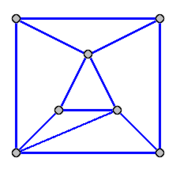An irregular polyhedron which has no automorphisms at all.

Given the amount of attention lavished on regular polytopes, one might almost think that all polytopes are regular. In reality, regular polytopes are just very special cases.

The simplest irregular polytope is the square pyramid, though this still has many symmetries.

An example of a polyhedron with no nontrivial symmetries is shown - no pair of vertices, edges, or 2-faces are "the same", as defined above. This is possibly the simplest such polytope.

## Realisations

Any traditional polytope is an example of a realisation of its underlying abstract polytope: The traditional pyramid to the left of the Hasse diagram above is a realisation of the poset represented. So also are tessellations or tilings of the plane, or other piecewise linear manifolds in two and higher dimensions. The latter include, for example, the projective polytopes. These can be obtained from a polytope with central symmetry by identifying opposite vertices, edges, faces and so forth. In three dimensions, this gives the hemi-cube and the hemi-dodecahedron, and their duals, the hemi-octahedron and the hemi-icosahedron.

More generally, a realisation of a regular abstract polytope is a collection of points in space (corresponding to the vertices of the polytope), together with the face structure induced on it by the polytope, which is at least as symmetrical as the original abstract polytope; that is, all combinatorial automorphisms of the abstract polytopes have been realized by geometric symmetries. For example, the set of points {(0,0), (0,1), (1,0), (1,1)} is a realisation of the abstract 4-gon (the square). It is not the only realisation, however - one could choose, instead, the set of vertices of a regular tetrahedron. For every symmetry of the square, there exists a corresponding symmetry of the regular tetrahedron. (There are, however, more symmetries of the regular tetrahedron than there are of the abstract 4-gon.)

In fact, every abstract polytope with v vertices has at least one realisation, as the vertices of a (v  1)-dimensional simplex. It is often of interest to seek lower-dimensional realisations.

If an abstract n-polytope is realized in n-dimensional space, such that the geometrical arrangement does not break any rules for traditional polytopes (such as curved faces, or ridges of zero size), then the realisation is said to be faithful. In general, only a restricted set of abstract polytopes of rank n may be realized faithfully in any given n-space. The characterisation of this effect is an outstanding problem.

## The amalgamation problem and universal polytopes

The basic theory of the combinatorial structures which are now known as "abstract polytopes" (but were originally called "incidence polytopes"), was first described in Egon Schulte's doctoral dissertation, although earlier work by Branko Grünbaum, H. S. M. Coxeter and Jacques Tits laid the groundwork. Since then, research in the theory of abstract polytopes has focused mostly on regular polytopes, that is, those whose automorphism groups act transitively on the set of flags of the polytope.

An important question in the theory of abstract polytopes is the amalgamation problem. This is a series of questions such as

For given abstract polytopes K and L, are there any polytopes P whose facets are K and whose vertex figures are L ?
If so, are they all finite ?
What finite ones are there ?

For example, if K is the square, and L is the triangle, the answers to these questions are

Yes, there are polytopes P with square faces, joined three per vertex (that is, there are polytopes of type {4,3}).
Yes, they are all finite, specifically,
There is the cube, with six square faces, twelve edges and eight vertices, and the hemi-cube, with three faces, six edges and four vertices.

It is known that if the answer to the first question is 'Yes' for some regular K and L, then there is a unique polytope whose facets are K and whose vertex figures are L, called the universal polytope with these facets and vertex figures, which covers all other such polytopes. That is, suppose P is the universal polytope with facets K and vertex figures L. Then any other polytope Q with these facets and vertex figures can be written Q=P/N, where

• N is a subgroup of the automorphism group of P, and
• P/N is the collection of orbits of elements of P under the action of N, with the partial order induced by that of P.

Q=P/N is called a quotient of P, and we say P covers Q.

Given this fact, the search for polytopes with particular facets and vertex figures usually goes as follows:

1. Attempt to find the applicable universal polytope
2. Attempt to classify its quotients.

These two problems are, in general, very difficult.

Returning to the example above, if K is the square, and L is the triangle, the universal polytope {K,L} is the cube (also written {4,3}). The hemicube is the quotient {4,3}/N, where N is a group of symmetries (automorphisms) of the cube with just two elements - the identity, and the symmetry that maps each corner (or edge or face) to its opposite.

If L is, instead, also a square, the universal polytope {K,L} (that is, {4,4}) is the tessellation of the Euclidean plane by squares. This tessellation has infinitely many quotients with square faces, four per vertex, some regular and some not. Except for the universal polytope itself, they all correspond to various ways to tessellate either a torus or an infinitely long cylinder with squares.

### The 11-cell and the 57-cell

The 11-cell, discovered independently by H. S. M. Coxeter and Branko Grünbaum, is an abstract 4-polytope. Its facets are hemi-icosahedra. Since its facets are, topologically, projective planes instead of spheres, the 11-cell is not a tessellation of any manifold in the usual sense. Instead, the 11-cell is a locally projective polytope. The 11-cell is not only beautiful in the mathematical sense, it is also historically important as one of the first non-traditional abstract polytopes discovered. It is self-dual and universal: it is the only polytope with hemi-icosahedral facets and hemi-dodecahedral vertex figures.

The 57-cell is also self-dual, with hemi-dodecahedral facets. It was discovered by H. S. M. Coxeter shortly after the discovery of the 11-cell. Like the 11-cell, it is also universal, being the only polytope with hemi-dodecahedral facets and hemi-icosahedral vertex figures. On the other hand, there are many other polytopes with hemi-dodecahedral facets and Schläfli type {5,3,5}. The universal polytope with hemi-dodecahedral facets and icosahedral (not hemi-icosahedral) vertex figures is finite, but very large, with 10006920 facets and half as many vertices.

### Local topology

The amalgamation problem has, historically, been pursued according to local topology. That is, rather than restricting K and L to be particular polytopes, they are allowed to be any polytope with a given topology, that is, any polytope tessellating a given manifold. If K and L are spherical (that is, tessellations of a topological sphere), then P is called locally spherical and corresponds itself to a tessellation of some manifold. For example, if K and L are both squares (and so are topologically the same as circles), P will be a tessellation of the plane, torus or Klein bottle by squares. A tessellation of an n-dimensional manifold is actually a rank n + 1 polytope. This is in keeping with the common intuition that the Platonic solids are three dimensional, even though they can be regarded as tessellations of the two-dimensional surface of a ball.

In general, an abstract polytope is called locally X if its facets and vertex figures are, topologically, either spheres or X, but not both spheres. The 11-cell and 57-cell are examples of rank 4 (that is, four-dimensional) locally projective polytopes, since their facets and vertex figures are tessellations of real projective planes. There is a weakness in this terminology however. It does not allow an easy way to describe a polytope whose facets are tori and whose vertex figures are projective planes, for example. Worse still if different facets have different topologies, or no well-defined topology at all. However, much progress has been made on the complete classification of the locally toroidal regular polytopes (McMullen & Schulte, 2002)

## Exchange maps

Let Ψ be a flag of an abstract n-polytope, and let −1 < i < n. From the definition of an abstract polytope, it can be proven that there is a unique flag differing from Ψ by a rank i element, and the same otherwise. If we call this flag Ψ(i), then this defines a collection of maps on the polytopes flags, say φi. These maps are called exchange maps, since they swap pairs of flags : (Ψφi)φi = Ψ always. Some other properties of the exchange maps :

• φi2 is the identity map
• The φi generate a group. (The action of this group on the flags of the polytope is an example of what is called the flag action of the group on the polytope)
• If |i  j| > 1, φiφj = φjφi
• If α is an automorphism of the polytope, then αφi = φiα
• If the polytope is regular, the group generated by the φi is isomorphic to the automorphism group, otherwise, it is strictly larger.

The exchange maps and the flag action in particular can be used to prove that any abstract polytope is a quotient of some regular polytope.

## Incidence matrices

A polytope can also be represented by tabulating its incidences. The following incidence matrix is that of a triangle:

øabcabbccaabc
ø
a
b
c
ab
bc
ca
abc

The table shows a dot wherever a face is a subface of another, or vice versa (so the table is symmetric about the diagonal)- so in fact, the table has redundant information; it would suffice to show only a dot when the row face ≤ the column face.

Since both the body and the empty set are incident with all other elements, the first row and column as well as the last row and column are trivial and can conveniently be omitted.

Further information is gained by counting each occurrence of an incidence as 1 (and hence non-incidence as 0). This numerative usage enables a symmetry grouping, as in the Hasse Diagram of the square pyramid: If vertices B, C, D, and E are considered symmetrically equivalent within the abstract polytope, then edges f, g, h, and j will be grouped together, and also edges k, l, m, and n, And finally also the triangles 'P', 'Q', 'R', and 'S'. Thus the corresponding incidence matrix of this abstract polytope may be shown as:

A  B,C,D,Ef,g,h,jk,l,m,nP,Q,R,S  T
A 1*4040
B,C,D,E *41221
f,g,h,j 114*20
k,l,m,n 02*411
P,Q,R,S 12214*
T 0404*1

In this accumulated incidence matrix representation the diagonal entries represent the total counts of either element type.

Elements of different type of the same rank clearly are never incident so the value will always be 0, however to help distinguish such relationships, an asterisk (*) is used instead of 0.

The sub-diagonal entries of each row represent the incidence counts of the relevant sub-elements, while the super-diagonal entries represent the respective element counts of the vertex-, edge- or whatever -figure.

Already this simple square pyramid shows that the symmetry-accumulated incidence matrices are no longer symmetrical. But there is still a simple entity-relation (beside the generalised Euler formulae for the diagonal, respectively the sub-diagonal entities of each row, respectively the super-diagonal elements of each row - those at least whenever no holes or stars etc. are considered), as for any such incidence matrixholds:## History

An early example of abstract polytopes was the discovery by Coxeter and Petrie of the three infinite structures {4, 6}, {6, 4} and {6, 6}, which they called regular skew apeirohedra.

In the 1960s Branko Grünbaum issued a call to the geometric community to consider generalizations of the concept of regular polytopes that he called polystromata. He developed a theory of polystromata, showing examples of new objects including the 11-cell.

The 11-cell is a self-dual 4-polytope whose facets are not icosahedra, but are "hemi-icosahedra" that is, they are the shape one gets if one considers opposite faces of the icosahedra to be actually the same face (Grünbaum, 1977). A few years after Grünbaum's discovery of the 11-cell, H.S.M. Coxeter discovered a similar polytope, the 57-cell (Coxeter 1982, 1984), and then independently rediscovered the 11-cell.

Egon Schulte defined "regular incidence complexes" and "regular incidence polytopes" in his PhD dissertation in the 1980s - the first time the modern definition was introduced. Subsequently, he and Peter McMullen developed the basics of the theory in a series of research articles that were later collected into a book. Numerous other researchers have since made their own contributions, and the early pioneers (including Grünbaum) had also accepted Schulte's definition as the "correct" one.